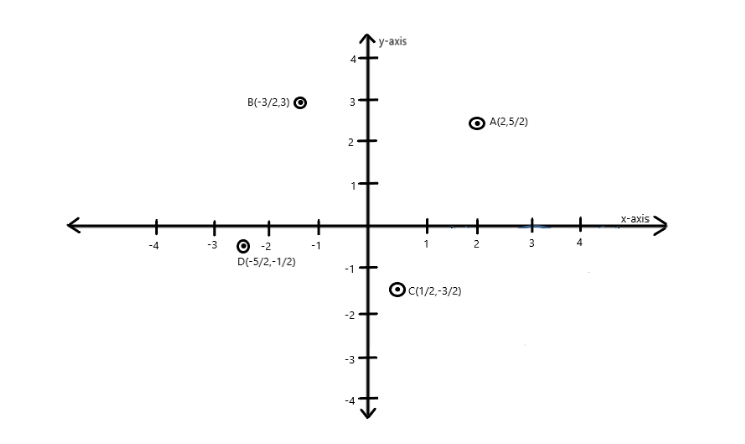# Plot the following points on the same graph paper: $A(2,\dfrac{5}{2}),\,B( - \dfrac{3}{2},3),\,C(\dfrac{1}{2},\dfrac{3}{2})\,and\,D( - \dfrac{5}{2}, - \dfrac{1}{2})$Verified
184.2k+ views
Hint: Coordinates are two numbers separated by a comma and they are always written in brackets. Coordinates are ordered pairs of numbers; the first number indicates the point on the x-axis and the second the point on the y-axis. A number on the x-axis is called x-coordinate. The x-coordinate is also called abscissa and a number on the y-axis is called y-coordinate. The y-coordinate is also called ordinate. For plotting points on a graph, we have to first identify the quadrant or axis in which the point lies.

For the point $A(2,\dfrac{5}{2})$ , the x-coordinate is $2$ and the y-coordinate is $\dfrac{5}{2}$ , both of the values are positive so point A lies in the first quadrant.
For the point $B( - \dfrac{3}{2},3)$ , the x-coordinate is $- \dfrac{3}{2}$ and the y-coordinate is $3$ , the value of x-coordinate is negative while that of y-coordinate is positive so point B lies in the second quadrant.
For the point $C(\dfrac{1}{2}, - \dfrac{3}{2})$ , the x-coordinate is $\dfrac{1}{2}$ and the y-coordinate is $- \dfrac{3}{2}$ , the value of y-coordinate is negative while that of x-coordinate is positive so point C lies in the fourth quadrant.
For the point $D( - \dfrac{5}{2}, - \dfrac{1}{2})$ , the x-coordinate is $- \dfrac{5}{2}$ and the y-coordinate is $- \dfrac{1}{2}$ , both of the values are negative so point D lies in the third quadrant.